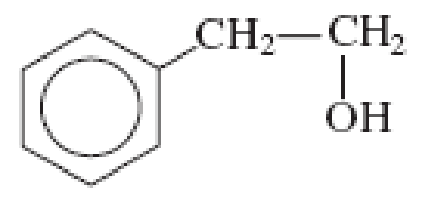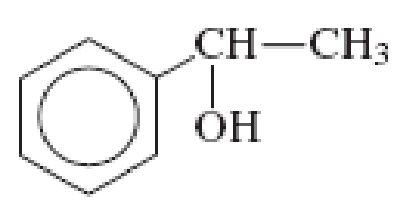Chapter 3, Problem 3.74EP### Organic And Biological Chemistry

7th Edition
STOKER + 1 other
ISBN: 9781305081079

#### Solutions

Chapter
Section### Organic And Biological Chemistry

7th Edition
STOKER + 1 other
ISBN: 9781305081079
Textbook Problem

# Indicate whether or not each of the following compounds is a phenol.(a)

Interpretation Introduction

Interpretation:

The given compound is phenol or not has to be indicated.

Concept Introduction:

Phenol is an aromatic compound.  This contains a hydroxyl group that is attached to an aromatic carbon ring system.  General formula of phenols is ArOH, where “Ar” represents aryl group.

Explanation

Given compound is,

Phenol is a compound that contains a hydroxyl group attached to an aryl group...

(b)

Interpretation Introduction

Interpretation:

The given compound is phenol or not has to be indicated.

Concept Introduction:

Phenol is an aromatic compound.  This contains a hydroxyl group that is attached to an aromatic carbon ring system.  General formula of phenols is ArOH, where “Ar” represents aryl group.

(c)

Interpretation Introduction

Interpretation:

The given compound is phenol or not has to be indicated.

Concept Introduction:

Phenol is an aromatic compound.  This contains a hydroxyl group that is attached to an aromatic carbon ring system.  General formula of phenols is ArOH, where “Ar” represents aryl group.

(d)

Interpretation Introduction

Interpretation:

The given compound is phenol or not has to be indicated.

Concept Introduction:

Phenol is an aromatic compound.  This contains a hydroxyl group that is attached to an aromatic carbon ring system.  General formula of phenols is ArOH, where “Ar” represents aryl group.

### Still sussing out bartleby?

Check out a sample textbook solution.

See a sample solution

#### The Solution to Your Study Problems

Bartleby provides explanations to thousands of textbook problems written by our experts, many with advanced degrees!

Get Started

#### Find more solutions based on key concepts